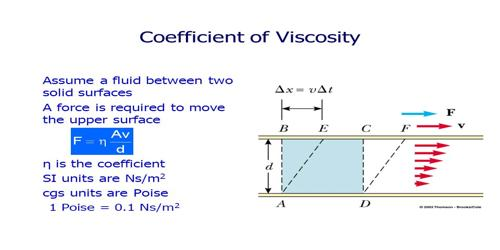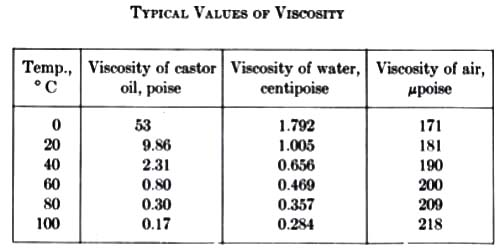# Effect of Temperature on Co-efficient of Viscosity in Gases

Effect of temperature on co-efficient of viscosity in Gases

The effect of temperature on gases is opposite to that of liquid. In case of gases co-efficient of viscosity increases with the increase of temperature. From experimental results it is seen that co-efficient of viscosity of gases is directly proportional to the square root of temperature i.e., η ∞ √T.

Explanation from kinetic theory of gases: We know, gas molecules move randomly in all directions and they collide with each other. The distance between molecules is much greater than the liquid molecules. So, intermolecular force between gas molecules is practically absent. When temperature increases average speed of molecules also increases and as a result a collision among molecules also increases. The gas viscosity will increase with temperature. According to the kinetic theory of gases, viscosity should be proportional to the square root of the absolute temperature, in practice, it increases more rapidly.Due to the increase of collisions resistance between the flows of different layers also increases i.e., viscosity also increases. The relation between average speed and temperature is,

c ∞ √T … … … (1)

Now, according to kinetic theory of gases, co-efficient of viscosity is proportional to average speed of the molecules. That means,

η ∞ c … … … (2)

From equations (1) and (2) we get,

η ∞ c ∞ √T

so, η ∞ √T

or, η = K √T

Here T is the temperature in Kelvin and K, the constant.